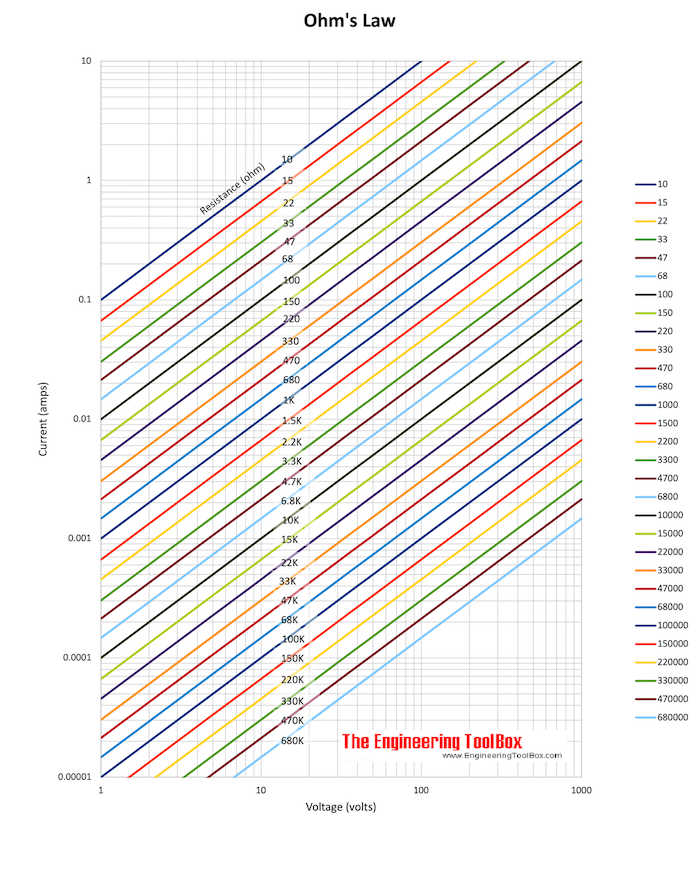Engineering ToolBox - Resources, Tools and Basic Information for Engineering and Design of Technical Applications!

# Ohm's Law

## The relation between voltage, current and electrical resistance.

Ohm's law states that

"the current through a conductor between two points is directly proportional to the potential difference or voltage across the two points, and inversely proportional to the resistance between them".

Ohm's law can be expressed as

I = U / R                     (1)

where

I = current (ampere, A)

U = electrical potential (volts, V)

R = resistance (ohms, Ω)

#### Example - Ohm's law

A 12 volt battery supplies power to a resistance of 18 ohms. The current in the elctrical circuit can be calculated as

I = (12 volts) / (18 ohm)

= 0.67 ampere### Equivalent Expressions of Ohm's Law

Ohm's law (1) can also be expressed as

U = R I                         (2)

or

R = U / I                       (3)#### Example - Electric Circuit Resistance

A current of 1 ampere is flowing through a 230 V electric circuit. From the diagram above this indicates resistance

R ≈ 220 Ω

This can alternatively be calculated with Ohm's law

R = (230 V) / (1 A)

= 230 Ω

#### Example - Ohm's Law and Multiples and Submultiples

Currents, voltages and resistances in electric circuits may often be very small or very large - so multiples and submultiples are often used.

The voltage required applied to a 3.3 kΩ resistor to generate a current of 20 mA can be calculated as

U = (3.3 kΩ) (1000 Ω/kΩ) (20 mA) (10-3 A/mA)

= 66 V

#### Electric Resistance Nomogram

The default values in the nomogram above indicates 230 volts, resistance 24 ohm and current 10 amps.

### Power

Electric power can be expressed as

P = U I

= R I2

= U2 / R                     (4)

where

P = electrical power (watts, W)

#### Example - Power Consumed

The power consumed in the 12V electrical circuit above can be calculated as

P = (12 volts)2 / (18 ohm)

=  8 W

#### Example - Power and Electrical Resistance

A 100 W electric light bulb is connected to a 230 V supply. The current flowing can be calculated by reorganizing (4) to

I = P / U

= (100 W) / (230 V)

= 0.43 ampere

The resistance can be calculated by reorganizing (4) to

R = U2 / P

= (230 V)2 / (100 W)

= 529 Ω

#### Electric Power Nomogram

This nomogram can be used to estimate power vs. voltage and ampere.

The default values in the nomogram above indicates 240 volts, resistance 10 amps and power 2.4 kW for DC or single phase AC - and 4 kW for three phase AC.

## Related Topics

• Electrical - Electrical units, amps and electrical wiring, wire gauge and AWG, electrical formulas and motors.

## Engineering ToolBox - SketchUp Extension - Online 3D modeling!

Add standard and customized parametric components - like flange beams, lumbers, piping, stairs and more - to your Sketchup model with the Engineering ToolBox - SketchUp Extension - enabled for use with the amazing, fun and free SketchUp Make and SketchUp Pro .Add the Engineering ToolBox extension to your SketchUp from the SketchUp Pro Sketchup Extension Warehouse!

Translate

## Privacy

We don't collect information from our users. Only emails and answers are saved in our archive. Cookies are only used in the browser to improve user experience.

Some of our calculators and applications let you save application data to your local computer. These applications will - due to browser restrictions - send data between your browser and our server. We don't save this data.

## Citation

• Engineering ToolBox, (2010). Ohm's Law. [online] Available at: https://www.engineeringtoolbox.com/ohms-law-d_1658.html [Accessed Day Mo. Year].

Modify access date.

. .

#### Scientific Online Calculator6 24